Next: 4. Oxidation of Doped Up: 3. Advanced Oxidation Model Previous: 3.2 Mathematical Formulation

# 3.3 Model Overview

The whole advanced oxidation model is based on a few main equations. The first one describes the oxidant diffusion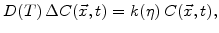(3.38)

and the next equation treats the dynamics ofwith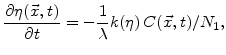(3.39)

as described in Section 3.2.1 and Section 3.2.2, respectively.

Because of the diffuse interface concept the volume increase of the generating oxide occurs only successively and not abruptly. As explained in Section 3.2.3, the volume increase of the oxidized material is calculated with theand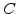values. After a time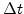the normalized additional volume is determined by(3.40)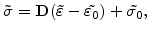(3.41)

because the principal axis components of the residual strain tensor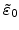are linearly proportional to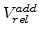in the form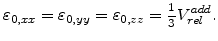(3.42)

The introduced so-called effective shear modulus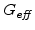in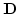(see Section 3.2.5.2) can handle elastic and visco-elastic materials.Next: 4. Oxidation of Doped Up: 3. Advanced Oxidation Model Previous: 3.2 Mathematical Formulation

Ch. Hollauer: Modeling of Thermal Oxidation and Stress Effects